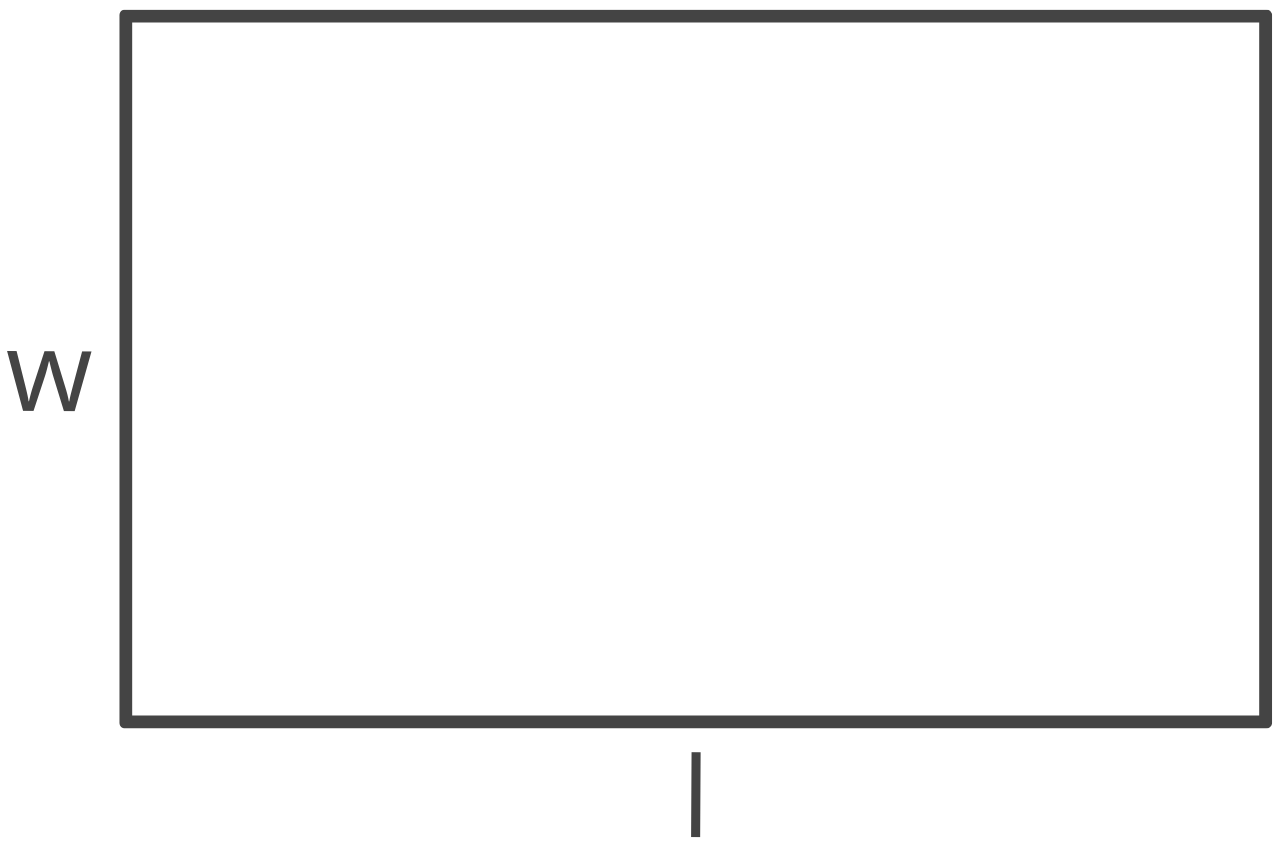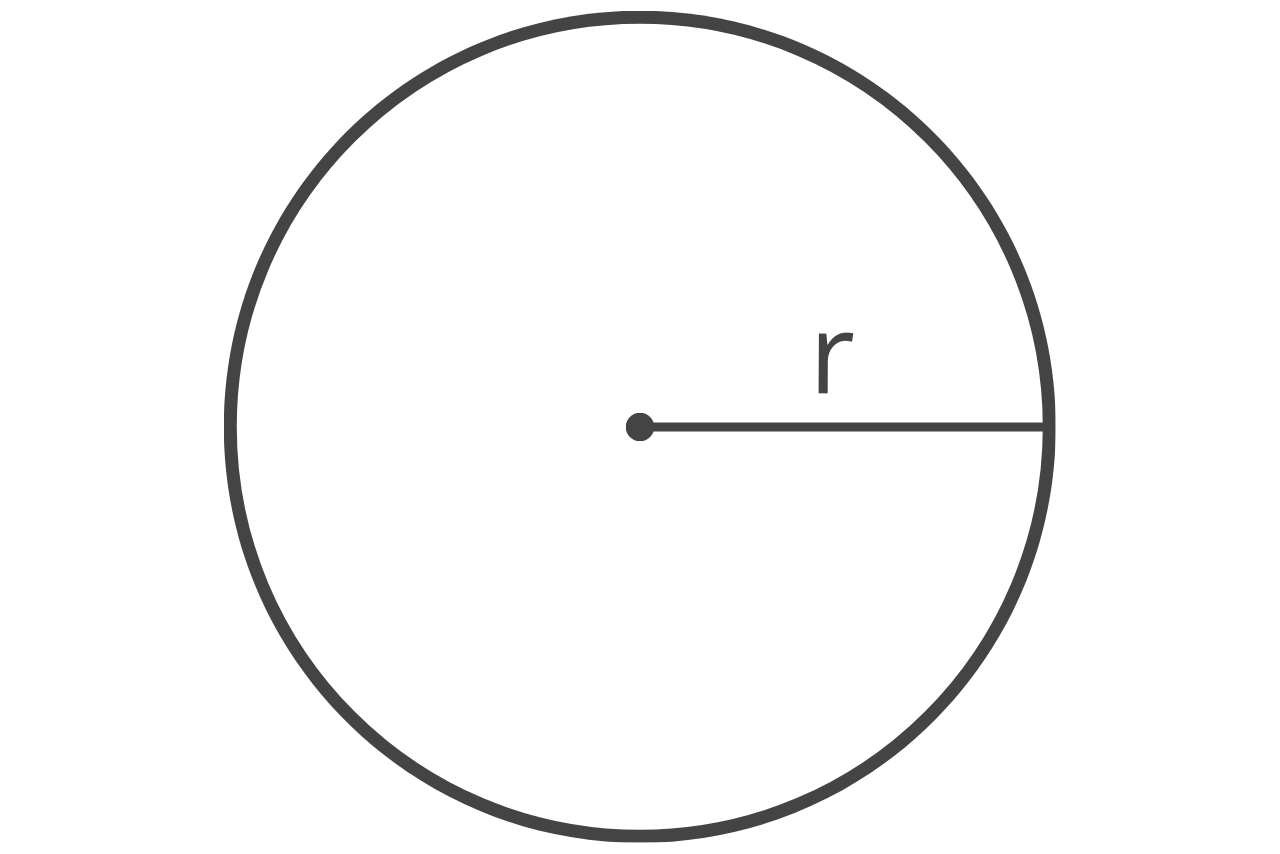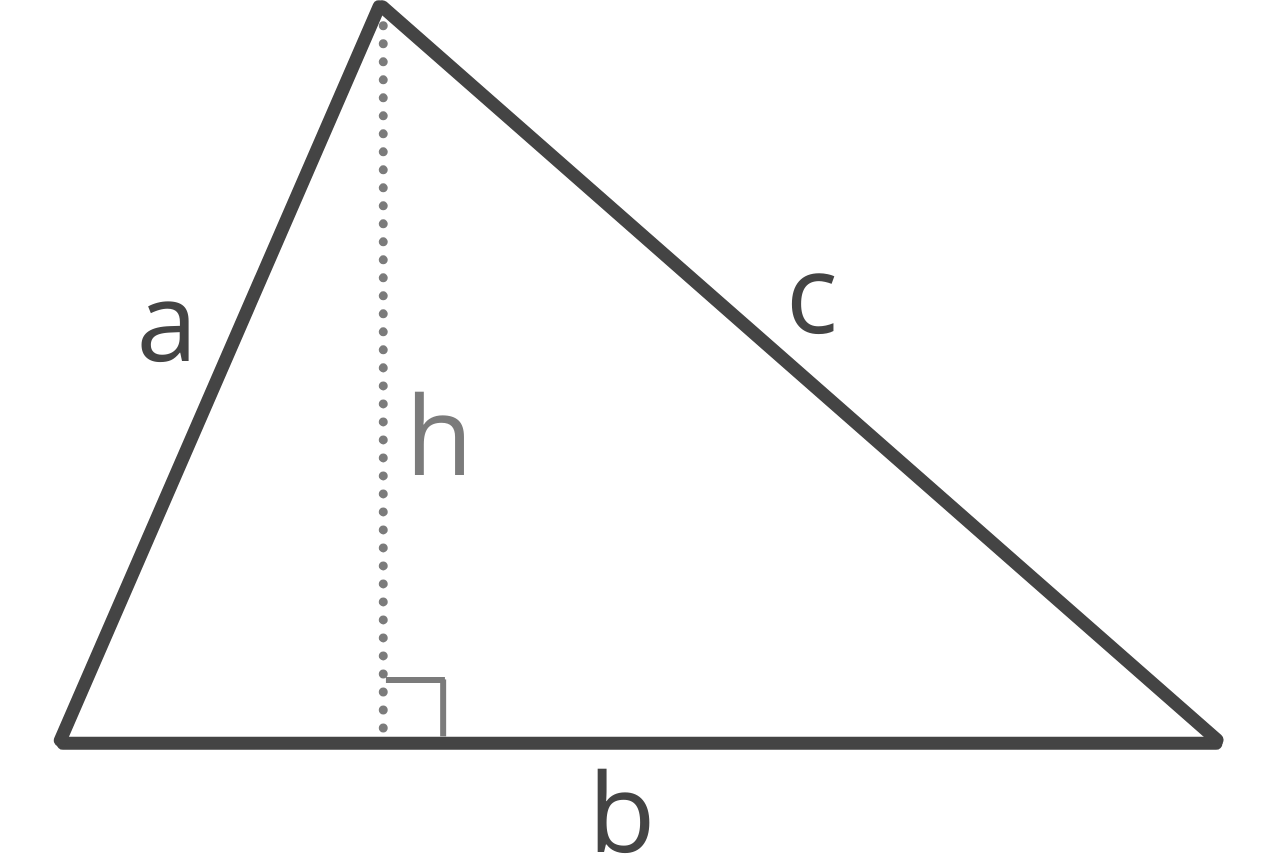# Square Inches Calculator

To calculate the area in square inches, enter the length and width in the calculator below.

## Results:

Sq In
Learn how we calculated this below

## How to Calculate Square Inches

Square inches are a unit of area measurement of a space. They are also referred to as inches squared. One square inch is equal to the area of a square with each side equal to one inch in length.

Of course, not all areas are perfect squares, but you can still calculate the area in square inches of a variety of shapes. For example, calculating the area of a rectangular shape can be done in a few easy steps.

### Step One: Measure the Length and Width

The first step to calculate the area of a rectangle in square inches is to measure the length and width of the area in inches using a ruler or tape measure.

If your measurement is in another unit of measure, such as centimeters, then you’ll need to convert to inches first. Use our length converter to convert your units to inches.

### Step Two: Multiply the Length and Width

The next step is to multiply the length and width to obtain the result in square inches. The square inches formula is:

square inches = length × width

Thus, the area is equal to length times width, both measured in inches. This is the same formula used to calculate square footage, except there the units of length and width are in inches rather than feet.

### Square Inches Formulas

If the area for which you are trying to calculate is not a rectangle, you can instead use the appropriate formula below to find the area in square inches for several shapes. Remember, in order for the area to have units of square inches, the dimensions of the shapes must be measured in inches.

#### Rectangle or Square

sq in = l × w

l = length
w = width#### Circle

sq in = πr2

π = 3.14159265359

Try our circle area calculator.#### Triangle

sq ft = bh/2

b = base
h = height

If you don’t know the height of the triangle, you can find it using the length of its sides.

s = 1/2(a + b + c)
sq in = s(s – a)(s – b)(s – c)

a = edge a
b = edge b
c = edge c

Try our triangle area calculator.You can use our area calculator to find the formulas for more shapes.